>Next: (c) Skewed Genomes Up: 2. Materials and Methods Previous: (iv) Variable Spacing

## (b) Formula for Rfrequency

If a genome contains G bases, there are M=G ways that its sequence can be aligned or G potential binding sites. If these are all equally likely, then Pi = 1/G and formula (1) reduces to: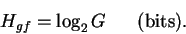(7)

If the genome contains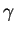sites, we assume that the probabilities of binding to each site are equal and that the probability of significant binding to other sequences is zero. This allows formula (1) to be reduced to: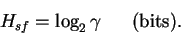(8)

(One property of H is that it is at a maximum when the probabilities are equal. Thus both Hgf and Hsf are maxima.)

The decrease in positional uncertainty during binding or alignment is the difference:

 Rfrequency =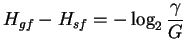=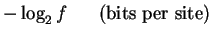(9)

where f is the frequency of sites in the genome.

Rfrequency is the amount of information needed to picksites out of G possible sites. As the number of sites in the genome increases, the information needed to find a site decreases. As long as the simplifying assumption for equation (8) holds andis restricted to the number of known sites (that is,is not an estimate), equation (9) gives an upper bound on Rfrequency since some sites may exist that are not now known. A second property of this formula is that Rfrequency is insensitive to small changes in G or. The frequency of sites must change by a factor of two to alter Rfrequency by only one bit. The largest possible value of Rfrequency occurs for a single site in the genome: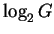. (For E. coli, Rfrequency = 22.9 bits in this case.) On the other hand, if all positions in the genome were sites, one would not need any information to find them, and Rfrequency would be zero.

The number of potential binding sites (G) is twice the number of base pairs in a DNA genome because there are two orientations for a recognizer to bind at each base pair. A symmetrical recognizer on DNA has two ways to bind each base pair, and both ways are used at a binding site. Here,is twice the number of conventional binding sites. An asymmetric recognizer on DNA will use only one orientation at any particular base pair. In this case,is equal to the number of binding sites. On RNA there is only one possible orientation. Thus G andreflect not only the genome size and number of binding sites but also the symmetries of the recognizer and nucleic acid.Next: (c) Skewed Genomes Up: 2. Materials and Methods Previous: (iv) Variable Spacing
Tom Schneider
2002-10-16